# Search

About 85 Search Results Matching Types of Worksheet, Worksheet Section, Generator, Generator Section, Subjects matching Math, Type matching Worksheet, Grades matching 4th Grade, Similar to Reading a Protractor Worksheet 2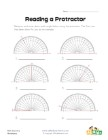## Reading a Protractor Worksheet 2

Read each protractor and write down the angles the...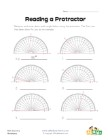## Reading a Protractor Worksheet 1

Read each protractor and write down the angles the...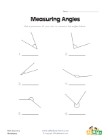## Measuring Angles Worksheet 2

Use a protractor to measure each of the angles.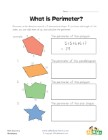## Perimeter Worksheet 2

Add up the length of the sides of each shape to fi...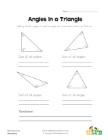## Angles in a Triangle Worksheet

Kids learn that the angles of a triangle always ad...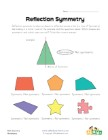## Symmetry Worksheet 2

Which shapes are symmetric and which are not?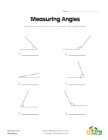## Measuring Angles Worksheet 1

Measure each of the angles with a protractor and w...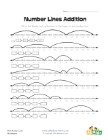## Number Lines Addition Worksheet 2

Fill in the blanks in the equations by using the n...